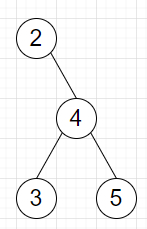# Program to traverse binary tree using list of directions in Python

Suppose we have a binary tree and a list of strings moves consisting of "R"(Right), "L"(Left) and "U"(Up). Starting from root, we have to traverse the tree by performing each move in moves where: "R" indicates traverse to the right child. "L" indicates traverse to the left child. "U" indicates traverse to its parent.

So, if the input is like["R","R","U","L"], then the output will be 3

To solve this, we will follow these steps −

• past := a new list

• for each move in moves, do

• insert root at the end of past

• if move is same as "L", then

• root := left of root

• otherwise when move is same as "R", then

• root := right of root

• otherwise,

• delete last element from past

• root := last element from past and delete it from past

• return value of root

Let us see the following implementation to get better understanding −

## Example

Live Demo

class TreeNode:
def __init__(self, data, left = None, right = None):
self.val = data
self.left = left
self.right = right
class Solution:
def solve(self, root, moves):
past = []
for move in moves:
past.append(root)
if move == "L":
root = root.left
elif move == "R":
root = root.right
else:
past.pop()
root = past.pop()
return root.val
ob = Solution()
root = TreeNode(2)
root.right = TreeNode(4)
root.right.left = TreeNode(3)
root.right.right = TreeNode(5)
traverse = ["R","R","U","L"]
print(ob.solve(root, traverse))

## Input

root = TreeNode(2) root.right = TreeNode(4) root.right.left =
TreeNode(3) root.right.right = TreeNode(5) ["R","R","U","L"]

## Output

3